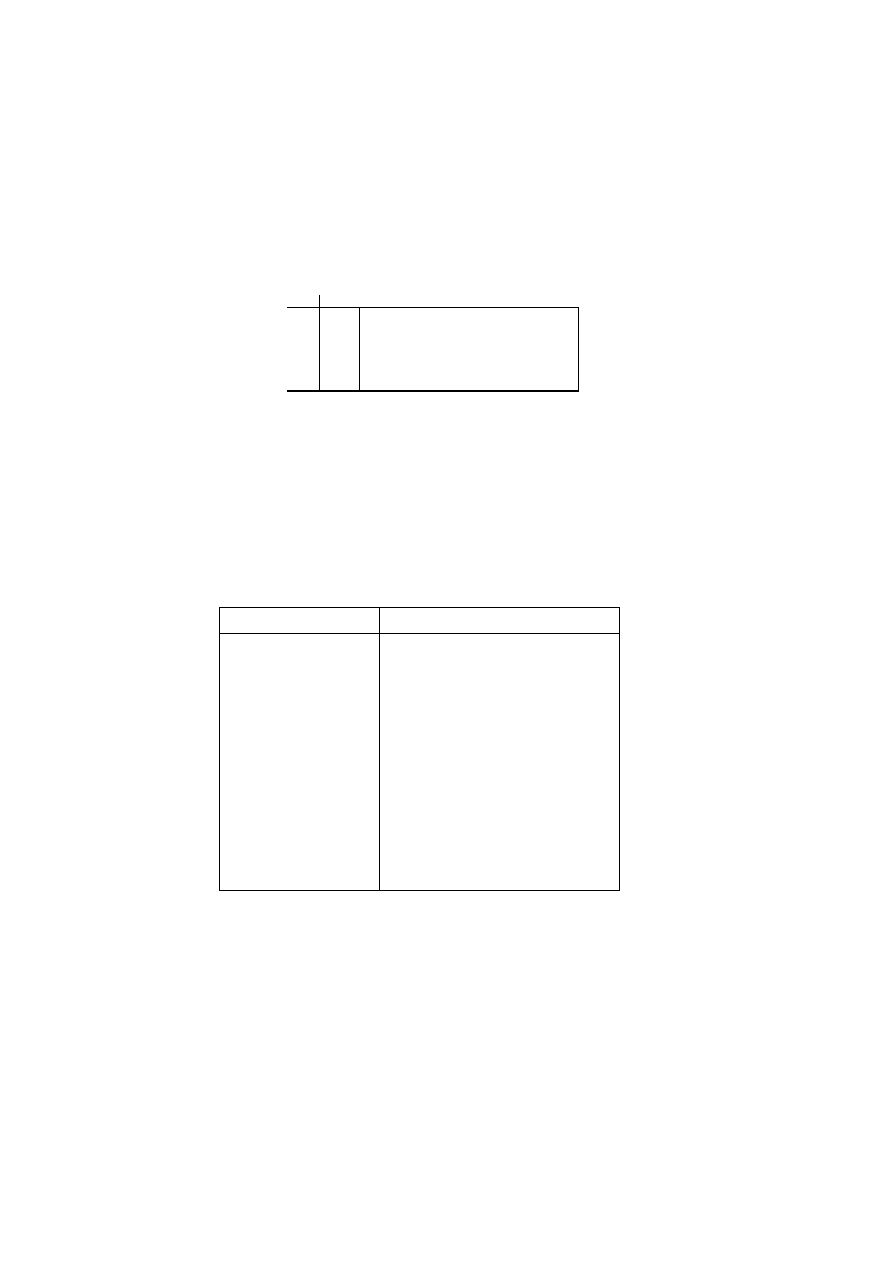ISO/IEC 10918-1 : 1993(E)
The 4 least significant bits, 'SSSS', define a category for the amplitude of the next non-zero coefficient in ZZ, and the 4
most significant bits, 'RRRR', give the position of the coefficient in ZZ relative to the previous non-zero coefficient (i.e.
the run-length of zero coefficients between non-zero coefficients). Since the run length of zero coefficients may exceed
=
X'F0' is defined to represent a run length of 15 zero coefficients followed by a coefficient
of zero amplitude. (This can be interpreted as a run length of 16 zero coefficients.) In addition, a special value
=
'00000000' is used to code the end-of-block (EOB), when all remaining coefficients in the block are
zero.
The general structure of the code table is illustrated in Figure F.1. The entries marked "N/A" are undefined for the
baseline procedure.
0
1
2
10
9
0
.
.
.
15
EOB
N/A
N/A
N/A
ZRL
. . .
RRRR
SSSS
TISO1330-93/d071
COMPOSITE VALUES
Figure F.1 ­ Two-dimensional value array for Huffman coding
Figure F.1 [D71] =4 cm = 156 %
The magnitude ranges assigned to each value of SSSS are defined in Table F.2.
Table F.2 ­ Categories assigned to coefficient values
SSSS
AC coefficients
1
1
­1,1
1
2
­3,­2,2,3
1
3
­7..­4,4..7
1
4
­15..­8,8..15
1
5
­31..­16,16..31
1
6
­63..­32,32..63
1
7
­127..­64,64..127
1
8
­255..­128,128..255
1
9
­511..­256,256..511
10
­1 023..­512,512..1 023
The composite value, RRRRSSSS, is Huffman coded and each Huffman code is followed by additional bits which specify
the sign and exact amplitude of the coefficient.
The AC code table consists of one Huffman code (maximum length 16 bits, not including additional bits) for each
possible composite value. The Huffman codes for the 8-bit composite values are generated in such a way that no code
consists entirely of 1-bits.
90
CCITT Rec. T.81 (1992 E)